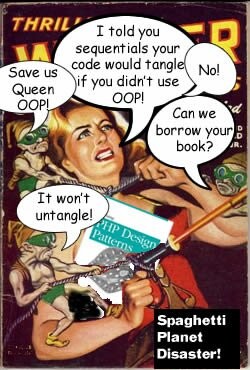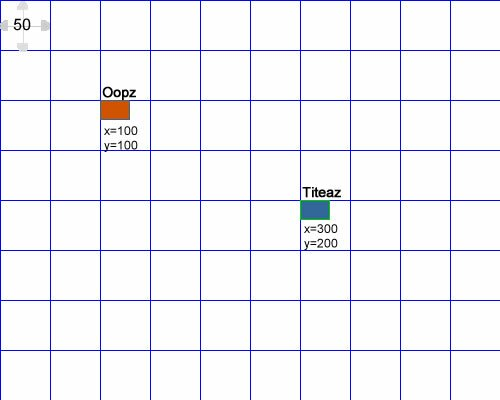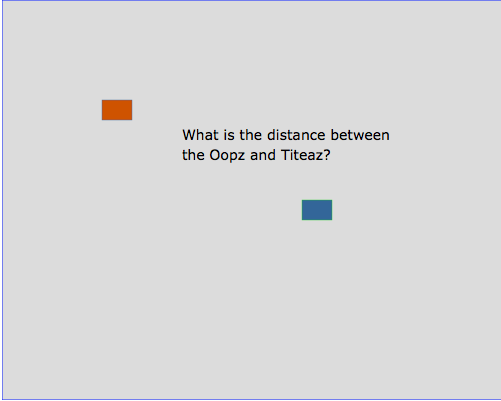PHP OOP Game Coding: Collision DetectionDistance in 2D Space

For a number of years I’ve had David Bourg’s book, Physics for Game Developers (2002, O’Reilly), and I’ve been meaning to translate a set of formulas into OOP classes that could be used as part of a PHP game development library. After spending time on (simple) game development last summer using Python, I decided it was time to get busy with a similar project using more OOP and PHP. I wanted something that was small enough to run on Raspberry Pi computers, but still an animated video game.

On previous posts on this blog I’ve used SVG graphics with PHP, but the examples I used were fairly static. Here I’d like to try them in a more dynamic role to see if PHP could generate code to make them dance. For starters I thought that a simple 2D space game would be appropriate — more on the order of Astroids than Space Aliens.

2D Outer Space on a Grid: Plane Geometry

In order to get anywhere, I decided that the universe (galaxy, solar system, whatever; you choose) would live on a 500 x 400 grid. It can be adjusted for different screens, but the first step is to set up a common grid for clear discussion. Further, I thought that starting with rectangles as ‘space ships’ would make everything else easier. (You can build something more elaborate later in the series.) The two space crafts are Oopz and Titeaz. Oopz is crewed by OOP developers, and Titeaz has a crew of sequential and procedural programmers who keep getting in trouble because of spaghetti knots and tight bindings. The Oopz goes on rescue missions to send them PHP code packages of classes and design patterns. Figure 1 shows the initial positions of the two ships:Figure 1: Grid with Oopz and Titeaz

Each of the grid squares in Figure 1 is 50 x 50 pixels, and the space ships use conventional a x|y position denotation.

Determining Distance and Collision Detection

The first thing we’ll tackle in Rocket Science 101 is determining the distance between two objects.

Raspberry Pi Users: You will need the Chromium browser for the graphics in this series. You can download it using the following code:
sudo apt-get install chromium

The distance between objects can be used for everything from determining when two objects have collided (distance = 0 + fudge-factor) to when another ship is in rescue range to receive project-saving OOP code. The SVG objects on your screen (without the grid) can be seen in Figure 2:Figure 2: Determining Distance

The code for this starting screen is based on the SVG W3 standards and saved as an XML file:



To see the distance calculation, click the Play button. See if you can figure out what formula is used before you look at the code:

The calculations are based on one of the most fundamental theorems in plane geometry. Before continuing, see if you can figure it out and resolve the solution.

Thank You Pythagoras!Figure 4: Pythagoras bust

If you concluded that it must be Pythagoras theorem used in the calculations, you are absolutely right. It’s one of those theorems that are so fundamental that we often overlook them for something more complex. The formula itself can be expressedwhere a and b are the shorter two sides of a right triangle and c (which is unknown in our case) is the longest side. As you saw in the Play sequence, we can figure out the positions of the two spaceships by their X and Y positions on the grid. By subtracting the X values from one another and the Y values from each other, we can get the values for a and b. All that’s left to be done is to put them into an algorithm encapsulated in a class. The Distance class returns the value of the distance based on the lengths of the shorter two legs of the right triangle formed on the grid.

 horizontal=\$h; \$this->vertical=\$v; \$this->distance=\$this->doGeom(); return \$this->distance; }   private function doGeom() { //Pythagorean theorem: // //Square of horizontal line \$this->horizontal = pow(\$this->horizontal,2);   //Square of the vertical line \$this->vertical=pow(\$this->vertical,2);   //The square root of the sum of the squares of //the other two sides of a right triangle //equal length of longest side = distance return sqrt(\$this->horizontal + \$this->vertical); } } ?>

If you take out all of the comments and OOP structure, the actual theorem can be boiled down to just three lines:

 ... \$this->horizontal = pow(\$this->horizontal,2); \$this->vertical=pow(\$this->vertical,2); return sqrt(\$this->horizontal + \$this->vertical); ...

This is pretty easy Rocket Science!

Finally, a Client class makes the request. I used a trigger script outside of the Client for the HTML call, but once a game is developed, the Client could be from any number of different clients as information is passed between objects.

 xpos=\$hor; \$this->ypos=\$vert; \$mapper = new Distance(); return \$mapper->getDistance(\$this->xpos,\$this->ypos); } } ?>

The trigger script creates a Client object, gets the super global values from HTML and then calls the appropriate method in the Client object to get the distance between the two objects. A more developed game would not require a trigger script and the value would not be printed to the screen but used to detect collisions.

 doDistance(\$hor,\$vert); ?>

Finally, the HTML script with the embedded SVG/XML code is only for demonstrations purposes, but you can open the following code to take a look at it if you want:

 Distance Calculator
Length of Horizontal line
Length of Vertical line

Green line's length

So What about Collision Detection?

The simplest collision detection occurs between two objects when their distance becomes zero. Essentially, the two objects have come to occupy the same space. As you saw from the example, if the coordinates of two objects on a grid are known, it is a matter of simple geometry to determine the distance between the two objects.

However, collision detection is more than shrinking distances and you can find doctoral dissertations that have been written on the subject. For the time-being, though, we can get started with 2D space and determining the distance in pixels between two objects. More robust methods of collision detection will have to wait until later.